# HSPT Math : How to do distance problems

## Example Questions

1 3 Next →

### Example Question #21 : How To Do Distance Problems

On a map, one half of an inch represents thirty miles of real distance.

The towns of Waterbury and Nashua are three and one half inches apart on this map. How long, in hours, would it take for someone to drive from Waterbury to Nashua if his speed averagedmiles per hour?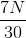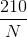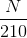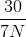Explanation:

On a map, one half of an inch represents thirty miles of real distance, so one inch represents twice this, or sixty miles. The actual distance from Waterbury to Nashua, which is three and a half inches on the map, is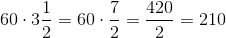Therefore, the two cities are 210 miles apart. Divide the distance by the rate to get the time: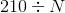, or.

### Example Question #36 : Word Problems

John is driving to his parents house on the highway going 45 mph. If he travels for 6 hours, how long was the trip?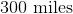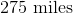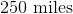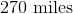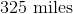Explanation:

You will use the distance formula of,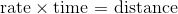.

Identify the rate and time that is given.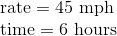From here, substitute the known information into the formula to calculate distance.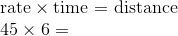First to find the ones digit multiply the ones digits together.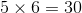Carry the three to the tens digit. Now, to find the tens and hundreds digit, multiply six by four and then add in the three that was carried.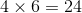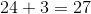Placing these numbers in their appropriate places results in the following solution.1 3 Next →